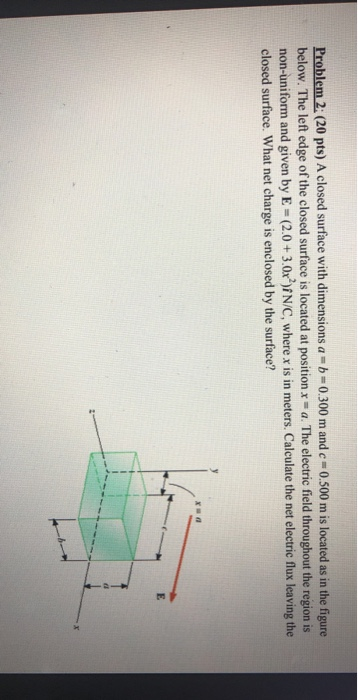# Question Solved1 AnswerProblem 2. (20 pts) A closed surface with dimensions a = b =0.300 m and e=0.500 m is located as in the figure below. The left edge of the closed surface is located at position x=a. The electric field throughout the region is non-uniform and given by E-(2.0+ 3.0x){N/C, where x is in meters. Calculate the net electric flux leaving the closed surface. What net charge is enclosed by the surface?Transcribed Image Text: Problem 2. (20 pts) A closed surface with dimensions a = b =0.300 m and e=0.500 m is located as in the figure below. The left edge of the closed surface is located at position x=a. The electric field throughout the region is non-uniform and given by E-(2.0+ 3.0x){N/C, where x is in meters. Calculate the net electric flux leaving the closed surface. What net charge is enclosed by the surface?
More
Transcribed Image Text: Problem 2. (20 pts) A closed surface with dimensions a = b =0.300 m and e=0.500 m is located as in the figure below. The left edge of the closed surface is located at position x=a. The electric field throughout the region is non-uniform and given by E-(2.0+ 3.0x){N/C, where x is in meters. Calculate the net electric flux leaving the closed surface. What net charge is enclosed by the surface?